# 含热粘性损耗的声学拓扑优化

2018年 2月 28日

### 标准声学拓扑优化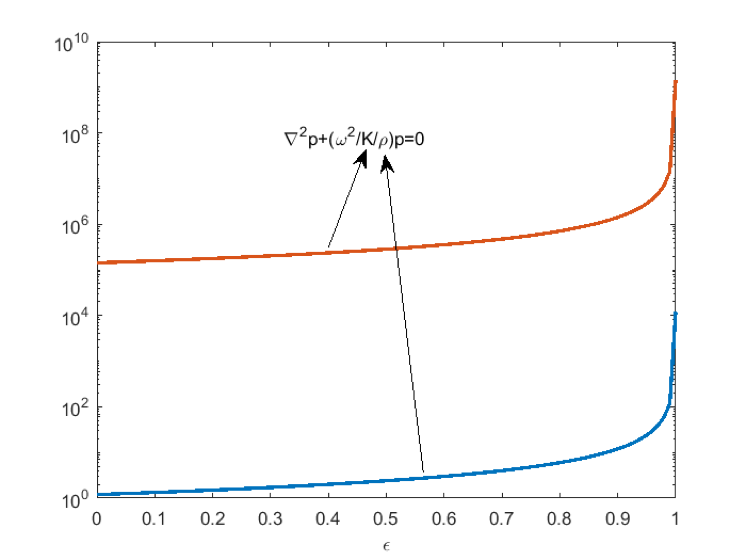#### 热粘性声学（微观声学）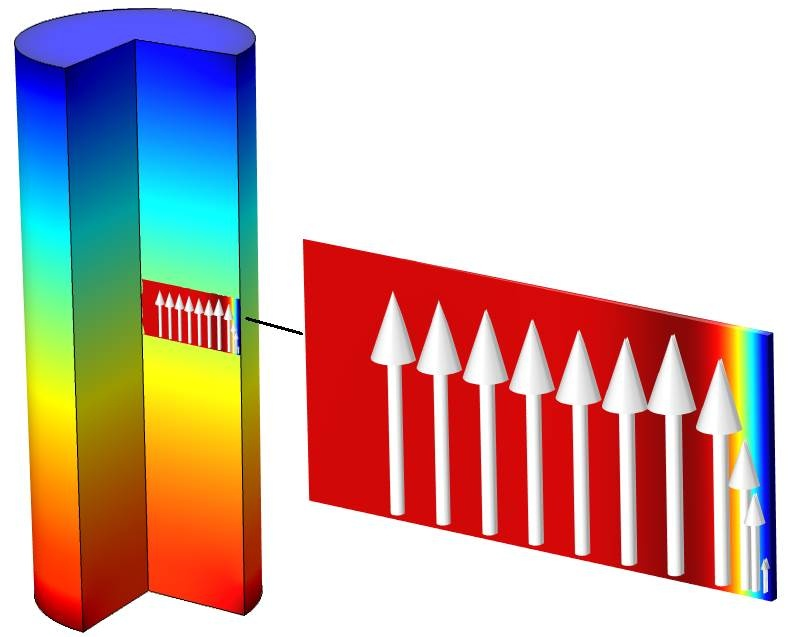### 热粘性声学控制方程

\vec{v}=\Psi_{v} \frac{\nabla{p}}{ik{\rho_0}c}

T=\Psi_{h} \frac{p}{{\rho_0}{C_p}}

### 热粘性声学应用的拓扑优化

(1)

\Psi_{v}+ k_v^{-2} \Delta_{cd} \Psi_{v}=1

\Psi_{v} = 0 \thickspace (no slip)

\nabla_{cd}\Psi_{v} = 0 \thickspace (slip)

a_{v} \Psi_{v}+k_{v}^{-2}\Delta_{cd}\Psi_{v}=f_{v}

a_{v} \Psi_{v} = 0

a_{v}(\epsilon)= \left\{ \begin{array}{ll}1\ \textrm{for}\ \epsilon=o \thickspace(air) \\ large\ \textrm{for} \ \epsilon=1 \thickspace (solid) \end{array} \right.

f_{v}(\epsilon)= \left\{ \begin{array}{ll}1\ \textrm{for}\ \epsilon=o \thickspace (air) \\ 0\ \textrm{for} \ \epsilon=1\thickspace (solid) \end{array} \right.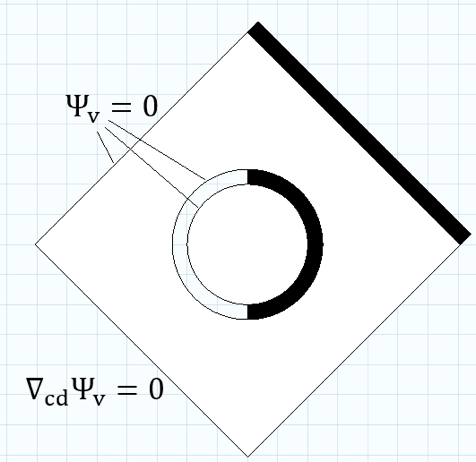### 优化损耗声学响应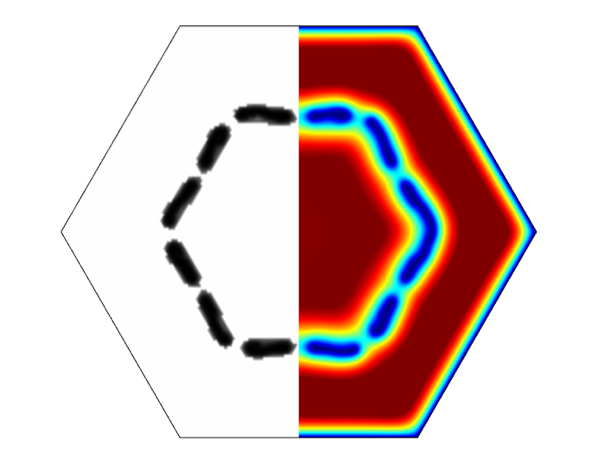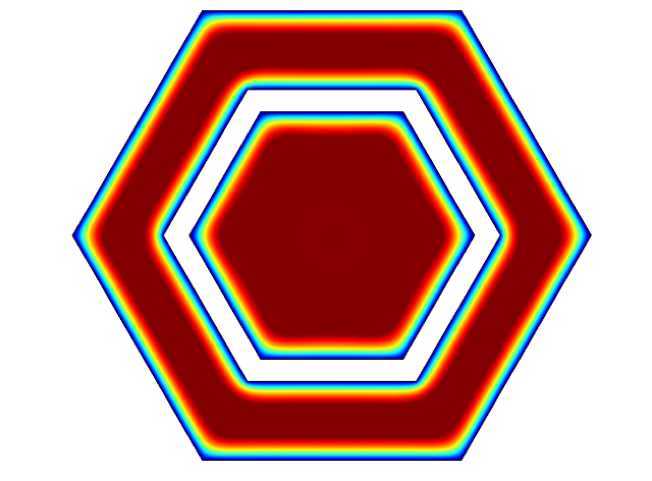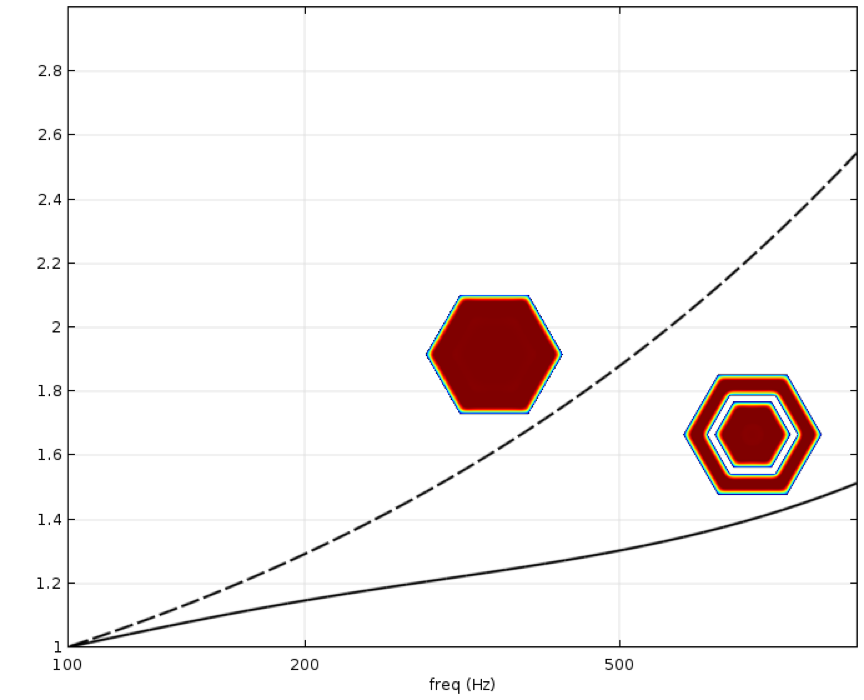### 参考文献

1. W.R. Kampinga, Y.H. Wijnant, A. de Boer, “An Efficient Finite Element Model for Viscothermal Acoustics,” Acta Acoustica united with Acoustica, vol. 97, pp. 618–631, 2011.
2. M.P. Bendsoe, O. Sigmund, Topology Optimization: Theory, Methods, and Applications, Springer, 2003.

### 特邀作者简介

René Christensen 在振动噪声领域拥有十多年工作经验，曾担任顾问（iCapture ApS）和助听器行业的工程师（Oticon A/S，GN Hearing A/S）。他对微观声学中的粘热效应的建模尤其感兴趣，这也是他的博士研究课题。2015 年，René 作为高级声学工程师加入了 GN Hearing 公司的硬件平台研发声学团队，主要负责助听器的设计和优化。